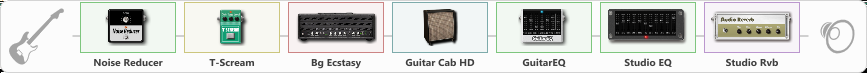# Rammstein Live Aus Berlin v.2

Discussion in 'ToneLib-GFX presets' started by Vallran, May 8, 2023.

1. Rammstein Live Aus Berlin v.2

Preset name: Rammstein Live Aus Berlin

Effects chain:Effect: "Noise Reducer" (Dynamics / Filter), active - "yes"
{
"Sens" = 56
"Mode" = Soft
}

Effect: "T-Scream" (Overdrive / Distortion), active - "yes"
{
"Drive" = 20
"Tone" = 71
"Level" = 100
}

Effect: "Bg Ecstasy" (Amp simulators), active - "yes"
{
"Gain" = 35
"Bass" = 32
"Middle" = 26
"Treble" = 42
"Presence" = 94
"Master" = 100
"Level (dB)" = 1
}

Effect: "Guitar Cab HD" (Cabinets), active - "yes"
{
"Model" = Bogner Ecstasy (4x12")
"Mic Model" = Condenser C414
"Mic Position" = Center
"Mic Distance" = Near
"Low Cut (Hz)" = 69
"Hi Cut (kHz)" = 20.0
"Mix" = 100
"Level (dB)" = 5
}

Effect: "GuitarEQ" (Dynamics / Filter), active - "yes"
{
"160 Hz" = -3
"400 Hz" = 0
"800 Hz" = -3
"1.6 kHz" = 0
"3.2 kHz" = -1
"6.4 kHz" = -6
"12 kHz" = -2
"Level (dB)" = 0
}

Effect: "Studio EQ" (Dynamics / Filter), active - "yes"
{
"31 Hz" = 0
"62 Hz" = 0
"125 Hz" = 0
"250 Hz" = 0
"500 Hz" = -7
"1 kHz" = -6
"2 kHz" = 0
"4 kHz" = 0
"8 kHz" = -1
"16 kHz" = -2
"Level (dB)" = 0
}

Effect: "Studio Rvb" (Reverberation), active - "yes"
{
"Time" = 5.5
"PreDelay" = 4
"LoDamp" = 0
"HiDamp" = 0
"Mix" = 53
}

Note: You will need to download and install the ToneLib-GFX software to use the preset.

#### Attached Files:

• ###### Rammstein_Live_Aus_Berlin_v2.tlgfx
File size:
763 bytes
Views:
796
psychonaut likes this.